﻿ Logarithmic Functions: Review and Additional Properties

# LOGARITHMIC FUNCTIONS: REVIEW AND ADDITIONAL PROPERTIES

LESSON READ-THROUGH (Part 1 of 2)
by Dr. Carol JVF Burns (website creator)
Follow along with the highlighted text while you listen!
• PRACTICE (online exercises and printable worksheets)

A logarithmic function is a function of the form $\,f(x) = \log_b x\,$ for $\,b > 0\,$ and $\,b\ne 1\,.$
The expression ‘$\,\log_b x\,$’ is read aloud as ‘log base $\,b\,$ of $\,x\,$’;
‘$\,\log_b\,$’ (read aloud as ‘log base $\,b\,$’) is the name of the function, and it acts on the input $\,x\,.$

When there's no confusion about order of operations,
mathematical convention dictates that we can drop the parentheses used to ‘hold’ the input,
and write ‘$\,\log_b x\,$’ (without parentheses) instead of the more cumbersome ‘$\,\log_b(x)\,$’.

If order of operations becomes ambiguous, be sure to include parentheses for clarification.
Thus, for example, you should write either $\,\log_b(x + 1)\,$ or $\,(\log_b x) + 1\,,$ but never the ambiguous ‘$\,\log_b x + 1\,$’.
(Notice, however, that there is no ambiguity in the expression ‘$\,1 + \log_b x\,$’.)

The number $\,b\,$ in $\,\log_b x \,$ is called the base of the logarithm.
When the base is $\,\text{e}\,,$ the function is called the natural logarithm, and can be written as $\,y = \ln x\,.$
(Note: $\text{e}\approx 2.71828\,$)

When the base is $\,10\,,$ the function is called the common logarithm, and can be written as $\,y = \log x\,.$

Logarithmic functions and their graphs were introduced in the Algebra II curriculum:

Additional higher-level information that is important for Precalculus is presented below.

## Increasing/Decreasing Properties of Logarithmic Functions

 When $\,b > 1\,,$ $\,f(x) = \log_b x\,$ is an increasing function. That is, for all positive real numbers $\,x\,$ and $\,y\,$: $$\cssId{s23}{x < y \ \ \Rightarrow\ \ \log_b x < \log_b y}$$ It is clear from the graph that the other direction is also true: $$\cssId{s25}{\log_b x < \log_b y \ \ \Rightarrow\ \ x < y}$$ Together, we have that for all positive real numbers $\,x\,$ and $\,y\,,$ and for $\,b > 1\,$: $$\cssId{s27}{x < y\ \ \text{is equivalent to}\ \ \log_b x < \log_b y} \tag{1}$$ Notice that when the base of the logarithm is greater than one, the inequality symbols that compare the inputs ($\,x\,$ and $\,y\,$) and their corresponding outputs ($\,\log_b x\,$ and $\,\log_b y\,$) have the same direction: $$\cssId{s31}{x \ {\bf\color{red}{<}}\ y\ \ \text{is equivalent to}\ \ \log_b x \ {\bf\color{red}{<}}\ \log_b y} \tag{1a}$$ This equivalence can be alternatively stated as: $$\cssId{s33}{x \ {\bf\color{red}{>}}\ y\ \ \text{is equivalent to}\ \ \log_b x\ {\bf\color{red}{>}}\ \log_b y} \tag{1b}$$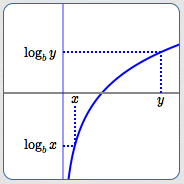$y = \log_b x\,,$ for $\,b > 1\,$ an increasing logarithmic function $x < y \ \ \iff\ \ \log_b x < \log_b y$ When $\,0 < b < 1\,,$ $\,f(x) = \log_b x\,$ is a decreasing function. That is, for all positive real numbers $\,x\,$ and $\,y\,$: $$\cssId{s40}{x < y \ \ \Rightarrow\ \ \log_b x > \log_b y}$$ It is clear from the graph that the other direction is also true: $$\cssId{s42}{\log_b x > \log_b y \ \ \Rightarrow\ \ x < y}$$ Together, we have that for all positive real numbers $\,x\,$ and $\,y\,,$ and for $\,0 < b < 1\,$: $$\cssId{s44}{x < y\ \ \text{is equivalent to}\ \ \log_b x > \log_b y} \tag{2}$$ Notice that when the base of the logarithm is between zero and one, the inequality symbols that compare the inputs ($\,x\,$ and $\,y\,$) and their corresponding outputs ($\,\log_b x\,$ and $\,\log_b y\,$) have different directions: $$\cssId{s48}{x \ {\bf\color{red}{<}}\ y\ \ \text{is equivalent to}\ \ \log_b x \ {\bf\color{red}{>}}\ \log_b y} \tag{2a}$$ This equivalence can be alternatively stated as: $$\cssId{s50}{x \ {\bf\color{red}{>}}\ y\ \ \text{is equivalent to}\ \ \log_b x \ {\bf\color{red}{<}}\ \log_b y} \tag{2b}$$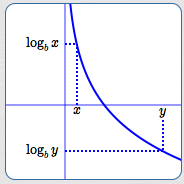$y = \log_b x\,,$ for $\,0 < b < 1\,$ a decreasing logarithmic function $x < y \ \ \iff\ \ \log_b x > \log_b y$

## Logarithmic Functions are One-to-One

The graphs of logarithmic functions pass both vertical and horizontal lines tests, so they are one-to-one functions.
Thus, for all positive real numbers $\,x\,$ and $\,y\,$: $$\cssId{s59}{x = y \ \ \text{is equivalent to}\ \ \log_b x = \log_b y} \tag{3}$$ Consequently, logarithmic functions have inverses.

## Logarithmic Functions and Exponential Functions are Inverses

Logarithmic functions and exponential functions with the same bases are inverses—they ‘undo’ each other.
Roughly:

• If the exponential function with base ‘$\,a\,$’ does something, then the logarithmic function with base ‘$\,a\,$’ undoes it.
• If the logarithmic function with base ‘$\,a\,$’ does something, then the exponential function with base ‘$\,a\,$’ undoes it.
These relationships are firmed up mathematically below:

 Drop $\,x\,$ in the   ‘exponential function with base $\,a\,$’   box;   get $\,y\,$ out the bottom. (See the top box in the diagram at right.) This is expressed mathematically as: $$\cssId{s71}{y = a^x}$$ Drop $\,y\,$ in the   ‘logarithmic function with base $\,a\,$’   box;   get $\,x\,$ out the bottom. (See the bottom box in the diagram at right.) This is expressed mathematically as: $$\cssId{s76}{\log_a y = x}$$ Since the exponential and logarithmic functions with base $\,a\,$ are inverses, these two operations are equivalent: $$\cssId{s78}{y = a^x\ \ \iff\ \ \log_a y = x}$$ If one is true, so is the other. If one is false, so is the other. They are true and false at the same time. In other words: If the exponential function with base $\,a\,$ takes $\,x\,$ to $\,y\,,$ then the logarithmic function with base $\,a\,$ takes $\,y\,$ back to $\,x\,.$ If the logarithmic function with base $\,a\,$ takes $\,y\,$ to $\,x\,,$ then the exponential function with base $\,a\,$ takes $\,x\,$ back to $\,y\,.$ ‘$\,y = a^x\,$’ is called the exponential form of the equation. ‘$\log_a y = x\,$’ is called the logarithmic form of the equation.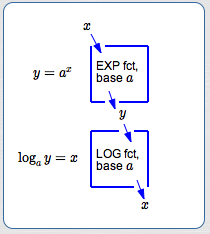The exponential and logarithmic functions with the same bases ‘undo’ each other! When ‘EXP fct, base $\,a\,$’ takes $\,x\,$ to $\,y\,,$ then ‘LOG fct, base $\,a\,$’ takes $\,y\,$ back to $\,x\,.$

## Going from Logarithmic Form to Exponential Form

Going from logarithmic form  ‘$\log_a y = x\,$’  to the equivalent exponential form  ‘$\,y = a^x\,$’  is easy!
Start with the base ‘$\,a\,$’, circle counterclockwise to $\,x\,,$ and then to $\,y\,$: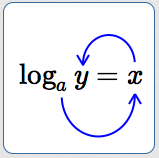This circular motion actually gives  ‘$\,a^x = y\,$’. Then, write it again from right-to-left to get ‘$\,y = a^x\,$’ !

## Going from Exponential Form to Logarithmic Form

Here's a thought process to go from exponential form  ‘$\,y = a^x\,$’  to the equivalent logarithmic form  ‘$\log_a y = x\,$’:

• We want a logarithmic form, so start by writing  $\,\color{red}{\log}\,$ .
• The base of the logarithm is the same as the base of the exponential function, so add in the base:  $\,\color{red}{\log_a}\,$ .
• A logarithm is an exponent!
The word ‘is’ translates as ‘$\,=\,$’.
The only exponent in  ‘$\,y = a^x\,$’  is $\,x\,.$
Leaving a space for the input, add in ‘equals $\,x\,$’:   $\,\color{red}{\log_a\ \ \ = x}\,$
• There's only one variable left in  ‘$\,y = a^x\,$’ , and only one place to put it!
Finish by putting in the  ‘$\,y\,$’ :   $\,\color{red}{\log_a y= x}\,$

## Domain/Range Considerations for Logarithmic and Exponential Functions

Let $\,a > 0\,,$ $\,a \ne 1\,.$ These are the allowable bases for logarithmic and exponential functions.

For exponential functions, $\,y = a^x\,$:

• the domain is the set of all real numbers
• the range is the set of positive real numbers
For logarithmic functions, $\,y = \log_a x\,$:
• the domain is the set of positive real numbers
• the range is the set of all real numbers
Observe that:
• the domain of $\,y = a^x\,$ is the range of $\,y = \log_a x\,$
• the range of $\,y = a^x\,$ is the domain of $\,y = \log_a x\,$
The input/output sets for inverses are switched!
The input set for one is the output set for the other.
The output set for one is the input set for the other.

## Two Important Views of Logarithms

Now, you should be comfortable with both important views of logarithms:

•   What is $\,\log_a x\,$?
It is the power (the exponent) that ‘$\,a\,$’ must be raised to, in order to get $\,x\,.$
• logarithms ‘undo’ exponents:
If the exponential function with base $\,a\,$ takes input $\,x\,$ to output $\,y\,,$ then what function takes $\,y\,$ back to $\,x\,$?
Answer: the logarithmic function with base $\,a\,$

## Solving Inequalities involving Logarithmic Functions

Equivalences (1), (2), and (3) can be used to solve certain types of mathematical sentences involving logarithms,
as illustrated in the following examples.

IMPORTANT: Whenever you have an expression of the form ‘$\,\log_a (\text{stuff})\,$’, the ‘stuff’ must be positive!

EXAMPLE 1:

Solve:

$\log_2(3x-1) < \log_2(5x)$
This is an inequality of the form $$\,\cssId{sb49}{\log_b(\text{stuff1}) < \log_b(\text{stuff2})}\,$$ where the base of the logarithm, $\,b\,,$ is $\,2\,.$

Notice that the logarithmic functions on both sides use the same base.

In this example, the base is greater than one, so we'll use equivalence (1): $$\cssId{sb53}{x < y \ \ \iff\ \ \log_b x < \log_b y}$$
SOLUTION:
\begin{alignat}{2} \log_2(3x-1) \ &< \ \log_2(5x)\qquad&&\text{original inequality}\cr\cr 3x-1 &< \ 5x&&\text{use (1); inequality symbol doesn't change}\cr\cr -2x \ &< 1&&\text{addition property for inequalities}\cr\cr x \ &> -\frac12&&\text{divide by a negative #; reverse inequality} \end{alignat}
Since logarithms only act on positive numbers, we must also have:
$$\begin{gather} 3x - 1 > 0 \quad \text{and} \quad 5x > 0\cr x > \frac 13 \quad \text{and} \quad x > 0 \end{gather}$$
Putting everything together: $$\cssId{sb59}{\bigl(x > -\frac 12 \ \ \text{and} \ \ x > \frac 13 \ \ \text{and} \ \ x > 0\bigr) \iff x > \frac 13}$$ The solution set of this inequality is: $$\cssId{sb61}{\underbrace{\{x\ |\ x > \frac 13\}}_{\text{set-builder notation}} = \underbrace{(\frac 13,\infty)}_{\text{interval notation}}}$$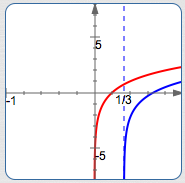blue curve:   $y = \log_2 (3x - 1)$ red curve:   $y = \log_2 (5x)$ The blue curve is only defined for $\,x > \frac 13\,.$ The blue curve lies below the red curve everywhere that it is defined.
Head up to wolframalpha.com and type in:

log_2 (3x -1) < log_2 (5x)

Voila!

 EXAMPLE 2: Solve: $\log_{0.3}(7) - \log_{0.3}(1 - 6x) > 0$ This is an inequality that can be easily transformed to $$\,\cssId{sb73}{\log_b(\text{stuff1}) > \log_b(\text{stuff2})}\,$$ where the base of the logarithm, $\,b\,,$ is $\,0.3\,.$ Notice that the logarithmic functions use the same base. In this example, the base is between zero and one, so we'll use equivalence (2): $$\cssId{sb77}{x < y \ \ \iff\ \ \log_b x > \log_b y}$$ Compare the solution at right to what you get at wolframalpha.com: log_(0.3) (7) - log_(0.3) (1 - 6x) > 0 SOLUTION: $$\begin{gather} \log_{0.3}(7) - \log_{0.3}(1 - 6x) > 0\cr\cr \log_{0.3}(7) > \log_{0.3}(1 - 6x)\cr\cr 7 < 1 - 6x \ \ \ \text{and}\ \ \ 1 - 6x > 0\cr\cr 6x < -6 \ \ \ \text{and}\ \ \ -6x > -1\cr\cr x < -1 \ \ \ \text{and}\ \ \ x < \frac 16\cr\cr x < -1 \end{gather}$$ Here are the reasons for each step: original inequality addition property for inequalities equivalence (2)—inequality symbol changes direction; inputs to logs must be positive addition property for inequalities multiplication property for inequalities How can a number be both less than $\,-1\,$ and less than $\,\frac 16\,$ at the same time? Answer: By being less than $\,-1\,$! Notice in the third step that the inequality symbol changed direction, since equivalence (2) is being used.

 EXAMPLE 3: Solve: $\ln(2x + 1)$ $\ \ = \ln(7x - 3)$ Here's a screenshot from wolframalpha.com: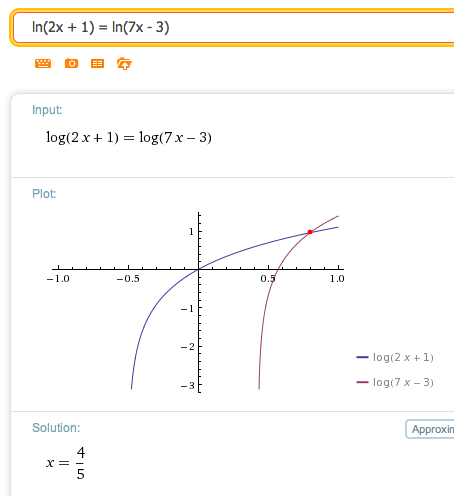Writing a list of equivalent mathematical sentences: $$\begin{gather} \ln(2x + 1) = \ln(7x - 3)\cr\cr 2x + 1 = 7x - 3 \ \ \text{and}\ \ 2x + 1 > 0 \ \ \text{and}\ \ 7x-3 > 0\cr\cr -5x = -4 \ \ \text{and}\ \ 2x > -1 \ \ \text{and}\ \ 7x > 3\cr\cr x = \frac 45 \ \ \ \text{and}\ \ \ x > -\frac 12 \ \ \ \text{and}\ \ \ x > \frac{3}{7}\cr\cr x = \frac 45 \end{gather}$$
Master the ideas from this section